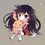# Solution to a Madoka Magica Problem

In chapter one of the anime series Maho Shojo Madoka Magica (roughly minute 13), the following problem is posed for middle school students as a whiteboard excercise:

Given $f(n) = \displaystyle{\frac{4n + \sqrt{4n^2 - 1}}{\sqrt{2n+1} + \sqrt{2n-1}}}$ find the sum of $f(1) + f(2) + f(3) + \dots + f(60)$

Akemi Homura, one of the main characters in the series, seems to solve it with some little algebraic mistakes here and there and following a fairly unconventional (although quite clever!) approach. I ended up thinking about this problem (as well as some other math problems shown in ths series and others) so much as to use it as a common excercise for my own students. So I wanted to share here the solution that (based on the calculations shown briefly on the series) I think is closest to the one Homura does:

Define $a_n = \sqrt{2n-1}$, that way $a_{n+1} = \sqrt{2(n+1)-1} = \sqrt{2n+1}$.

Note that $a_n \cdot a_{n+1} = \sqrt{(2n-1)(2n+1)} = \sqrt{4n^2 - 1}$

Now, we can write $4n$ as $(2n-1) + (2n + 1) = a_n^2 + a_{n+1}^2$ and the function $f(n)$ can be written in terms of this sequence as follows:

$f(n) = \displaystyle{\frac{a_n^2 + a_{n+1}^2 + a_n \cdot a_{n+1}}{a_n + a_{n+1}} = \frac{a_{n+1}^2 + a_n \cdot a_{n+1} + a_n^2}{a_{n+1} + a_n}}$

Multiplying up and down by $a_{n+1} - a_n$ and using the identities for difference of squares and cubes yields:

$f(n) = \displaystyle{\frac{a_{n+1}^3 - a_n^3}{a_{n+1}^2 - a_n^2}}$

As a last simplification, we note that $a_{n+1}^2 - a_n^2 = (2n+1) - (2n-1) = 2$ so the sum turns into:

$\displaystyle{\sum_{k=1}^{60} \frac{a_{k+1}^3 - a_k^3}{2}} = \frac{1}{2} \sum_{k=1}^{60} a_{k+1}^3 - a_k^3$

Which is a telescoping sum that evaluates to $a_{61}^3 - a_1^3 = (2 \cdot 61 - 1)^{3/2} - (2 \cdot 1 - 1)^{3/2} = 11^3 - 1 = 1330$

So multiplying by $\frac{1}{2}$ we have the final result:

$f(1) + f(2) + f(3) + \dots + f(60) = 665$Note by Benja Vera
1 year, 1 month ago

This discussion board is a place to discuss our Daily Challenges and the math and science related to those challenges. Explanations are more than just a solution — they should explain the steps and thinking strategies that you used to obtain the solution. Comments should further the discussion of math and science.

When posting on Brilliant:

• Use the emojis to react to an explanation, whether you're congratulating a job well done , or just really confused .
• Ask specific questions about the challenge or the steps in somebody's explanation. Well-posed questions can add a lot to the discussion, but posting "I don't understand!" doesn't help anyone.
• Try to contribute something new to the discussion, whether it is an extension, generalization or other idea related to the challenge.
• Stay on topic — we're all here to learn more about math and science, not to hear about your favorite get-rich-quick scheme or current world events.

MarkdownAppears as
*italics* or _italics_ italics
**bold** or __bold__ bold
- bulleted- list
• bulleted
• list
1. numbered2. list
1. numbered
2. list
Note: you must add a full line of space before and after lists for them to show up correctly
paragraph 1paragraph 2

paragraph 1

paragraph 2

[example link](https://brilliant.org)example link
> This is a quote
This is a quote
    # I indented these lines
# 4 spaces, and now they show
# up as a code block.

print "hello world"
# I indented these lines
# 4 spaces, and now they show
# up as a code block.

print "hello world"
MathAppears as
Remember to wrap math in $$ ... $$ or $ ... $ to ensure proper formatting.
2 \times 3 $2 \times 3$
2^{34} $2^{34}$
a_{i-1} $a_{i-1}$
\frac{2}{3} $\frac{2}{3}$
\sqrt{2} $\sqrt{2}$
\sum_{i=1}^3 $\sum_{i=1}^3$
\sin \theta $\sin \theta$
\boxed{123} $\boxed{123}$

## Comments

Sort by:

Top Newest

Is there any way i can share this on facebook? i find it hilarious and, at the same time, fairly interesting

- 1 year, 1 month ago

Log in to reply

ohhh I can't find a share button anywhere:( But you can paste the url if you want!

- 1 year, 1 month ago

Log in to reply

Nice!, @Benja Vera

- 1 year, 1 month ago

Log in to reply

×

Problem Loading...

Note Loading...

Set Loading...